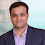# HOW TO READ DATA FROM EXCEL FILE (.XLS) USING APACHE POI?

We can read data from excel file using Apache POI library.
Here is the very basic program to explain How to read data from excel file using Apache POI library.

Create a testdata.xls file in the C drive with following details:

 Search Text hello selenium abhishek yadav qa

#### Java Source code:

```package com.helloselenium.selenium.test;

import java.io.*;

import org.apache.poi.hssf.usermodel.*;

public static void main(String[] args) {

try {

FileInputStream file = new FileInputStream(new File("C:\\testdata.xls"));
HSSFWorkbook workbook = new HSSFWorkbook(file);

HSSFSheet sheet = workbook.getSheetAt(0);

String searchText1 = sheet.getRow(1).getCell(0).getStringCellValue();

String searchText2 = sheet.getRow(2).getCell(0).getStringCellValue();

System.out.println("Search Text 1 is:" + searchText1);

System.out.println("Search Text 2 is:" + searchText2);

file.close();

} catch (FileNotFoundException fnfe) {
fnfe.printStackTrace();
} catch (IOException ioe) {
ioe.printStackTrace();
}
}
}
```

##### Output:
Search Text 1 is:Hello Selenium
Search Text 2 is:abhishek yadav qa

Detailed explanation for the above program is as follows:

Following code is the required packages for JAVA IO to make integration with excel file.
```import java.io.*;
```
Following code is the required packages for Apache POI library.
```import org.apache.poi.hssf.usermodel.*;
```
Following code is to locate the path of excel file.
```FileInputStream file = new FileInputStream(new File("C:\\testdata.xls"));
```
Following code is to initialize the excel file as a workbook.
```HSSFWorkbook workbook = new HSSFWorkbook(file);
```
Following code is to initialize the excel sheet of the workbook. Here 0 (zero) refers to the first sheet of the workbook.
```HSSFSheet sheet = workbook.getSheetAt(0);
```
Following code is to get the value from the worksheet. Here 0 (zero) refers to first row and first cell of the worksheet.
```String heading = sheet.getRow(0).getCell(0).getStringCellValue();
```
Following code is to get the value from the worksheet. Here 1 refers to second row and and 0 (zero) refers to first cell of the worksheet.
```String searchText1 = sheet.getRow(1).getCell(0).getStringCellValue();
```

Following code is to get the value from the worksheet. Here 2 refers to third row and 0 (zero) refers to first cell of the worksheet.
```String searchText2 = sheet.getRow(2).getCell(0).getStringCellValue();
```
Following code is to print the stored string variable in console.
```System.out.println("Heading is:" + heading);
```
Following code is to print the stored string variable in console.
```System.out.println("Search Text 1 is:" + searchText1);
```
Following code is to print the stored string variable in console.
```System.out.println("Search Text 2 is:" + searchText2);
```
You can also use the following code is to close the excel file.
```file.close();
```

1.How do i compare data from 2 excels
I have written below code however, it is not giving me the output.

clicsDriver.findElement(By.id("GenReportForm:fetchBtn")).click();
System.out.println("input for 3rd party report entered successfully");
data= new String[totalNoOfCols][totalNoOfRows];
dataTemplate= new String[totalNoOfColsTemplate][totalNoOfRowsTemplate];

//for generated report xls
try {
String FilePath = "C:\\Users\\A592019\\Desktop\\NSN\\CLicS Requirements\\CLicS 9.1 Test Data\\7196\\Copy of General_Report_2015_04_14_11_35_41.xls";
FileInputStream fs = new FileInputStream(FilePath);
workbook= Workbook.getWorkbook(fs);
sheet= workbook.getSheet(1);
totalNoOfRows = sheet.getRows();
totalNoOfCols = sheet.getColumns();

for (int i=0; i<= totalNoOfRows; i++)
{
for (int j=0; j<= totalNoOfCols; j++)
{
data[j][i]= sheet.getCell(j, i).getContents();
System.out.println("data array"+data[j][i]+"\t");
}
}
}
catch (BiffException e) {
// TODO Auto-generated catch block
e.printStackTrace();
}catch(IOException e){
}
//for stored report template All fields
try {
String FilePathTemplate = "C:\\Users\\A592019\\Desktop\\NSN\\CLicS Requirements\\CLicS 9.1 Test Data\\7196\\3rd party general report all fields.xls";
FileInputStream fsTemplate = new FileInputStream(FilePathTemplate);
workbook= Workbook.getWorkbook(fsTemplate);
sheet= workbook.getSheet(1);
totalNoOfRowsTemplate = sheet.getRows();
totalNoOfColsTemplate = sheet.getColumns();
//dataTemplate=new String[totalNoOfColsTemplate];

for (int i=0; i<= totalNoOfRowsTemplate; i++)
{
for (int j=0; j<= totalNoOfColsTemplate; j++)
{
dataTemplate[j][i]= sheet.getCell(j, i).getContents();
System.out.println("dataTemplate array"+dataTemplate[j][i]+"\t");
}
}
}
catch (BiffException e) {
// TODO Auto-generated catch block
e.printStackTrace();
}catch(IOException e){
}
//Compare data in report and the template
//int outputRows= Integer.parseInt(dataTemplate[totalNoOfRowsTemplate][totalNoOfColsTemplate]);

for (int row=0; row<= totalNoOfRowsTemplate; row++)
{
for (int cols=0; cols<= totalNoOfColsTemplate; cols++)
{
if (dataTemplate[cols][row] == data[cols][row])
{
System.out.println("Data matches");
}
else
{
System.out.println("Issue in the template. Report template does not match");
}
}
}
}
}

1.